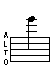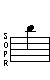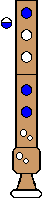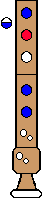Fingerings Home Octave 1: F  (C) Gb  (Db) G  (D) Ab  (Eb) A  (E) Bb  (F) B  (Gb) C  (G) Db  (Ab) D  (A) Eb  (Bb) E  (B) Octave 2+: F  (C) Gb  (Db) G  (D) Ab  (Eb) A  (E) Bb  (F) B  (Gb) C  (G) Db  (Ab) D  (A) Eb  (Bb) E  (B) F  (C) Gb  (Db) G  (D)E (B) - 2nd Octave## Trills

Trill - From Gb   Trill - From F
Gb E   F ETop

## Turned Trills

Turned Trill
Full step above, Full step below
Gb E D E

Top

Turned Trill
Half step above; Full step below
F E D (alt) ETop

Turned Trill
Full step above; Half step below
Gb (alt) E Eb E

Top

Turned Trill
Half step above; Half step below
F E Eb ETop

## Regular Turns

Turn
Full step above; Full step below
Gb E D EComments: For the Gb, you must cover the hole at the bell end of the recorder with your knee. This is very difficult, of course.
Top

Turn
Half step above; Full step below
F E D ETop

Turn
Full step above; Half step below
Gb E Eb EComments: For the Gb, you must cover the hole at the bell end of the recorder with your knee. This is very difficult, of course.
Top

Turn
Half step above; Half step below
F E Eb E